# Selected ATcT [1, 2] enthalpy of formation based on version 1.122p of the Thermochemical Network 

This version of ATcT results was generated from an expansion of version 1.122o  to include an updated enthalpy of formation for Hydrazine. .

Species Name Formula Image    ΔfH°(0 K)    ΔfH°(298.15 K) Uncertainty Units Relative
Molecular
Mass
ATcT ID
NitromethaneCH3N(O)O (cr,l)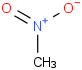-114.18-113.00± 0.43kJ/mol61.0401 ±
0.0010
75-52-5*500

## Top contributors to the provenance of ΔfH° of CH3N(O)O (cr,l)

The 14 contributors listed below account for 90.0% of the provenance of ΔfH° of CH3N(O)O (cr,l).

Please note: The list is limited to 20 most important contributors or, if less, a number sufficient to account for 90% of the provenance. The Reference acts as a further link to the relevant references and notes for the measurement. The Measured Quantity is normaly given in the original units; in cases where we have reinterpreted the original measurement, the listed value may differ from that given by the authors. The quoted uncertainty is the a priori uncertainty used as input when constructing the initial Thermochemical Network, and corresponds either to the value proposed by the original authors or to our estimate; if an additional multiplier is given in parentheses immediately after the prior uncertainty, it corresponds to the factor by which the prior uncertainty needed to be multiplied during the ATcT analysis in order to make that particular measurement consistent with the prevailing knowledge contained in the Thermochemical Network.

Contribution
(%)
TN
ID
Reaction Measured Quantity Reference
52.9CH3N(O)O (cr,l) + 3/2 O2 (g) → 2 CO2 (g) + 3 H2O (l) N2 (g) ΔrH°(298.15 K) = -339.02 ± 0.28 kcal/molProsen 1954, Cass 1958
25.9CH3N(O)O (cr,l) + 3/2 O2 (g) → 2 CO2 (g) + 3 H2O (l) N2 (g) ΔrH°(298.15 K) = -339.2 ± 0.4 kcal/molLebedeva 1973, note unc2
4.1CH3N(O)O (cr,l) + 3/2 O2 (g) → 2 CO2 (g) + 3 H2O (l) N2 (g) ΔrH°(298.15 K) = -339.0 ± 1 kcal/molSwientoslawski 1910, est unc
1.6CH3N(O)O (cr,l) + 3/2 O2 (g) → 2 CO2 (g) + 3 H2O (l) N2 (g) ΔrH°(298.15 K) = -1425.02 ± 1.10 (×6.037) kJ/molVerevkin 2014
1.1ΔrH°(0 K) = 12.170 ± 0.040 eVRuscic W1RO
0.6ΔrH°(0 K) = -1.91 ± 1.2 kcal/molRuscic W1RO
0.5ΔrH°(0 K) = -1.86 ± 1.3 kcal/molRuscic CBS-n
0.5ΔrH°(0 K) = -2.33 ± 1.3 kcal/molRuscic G4
0.4ΔrH°(0 K) = -1.77 ± 1.4 kcal/molRuscic G3X
0.4CH3N(O)O (cr,l) + 3/2 O2 (g) → 2 CO2 (g) + 3 H2O (l) N2 (g) ΔrH°(298.15 K) = -336.0 ± 0.6 (×5.076) kcal/molKnobel 1971
0.4CH3N(O)O (g) → C (g) + 3 H (g) N (g) + 2 O (g) ΔrH°(0 K) = 570.15 ± 1.50 kcal/molRuscic W1RO
0.4ΔrH°(0 K) = 59.79 ± 1.50 kcal/molRuscic W1RO
0.3CH3N(O)O (g) → C (g) + 3 H (g) N (g) + 2 O (g) ΔrH°(0 K) = 570.99 ± 1.60 kcal/molRuscic G4, Dorofeeva 2013
0.3ΔrH°(0 K) = 58.58 ± 1.60 kcal/molRuscic G4

## Top 10 species with enthalpies of formation correlated to the ΔfH° of CH3N(O)O (cr,l)

Please note: The correlation coefficients are obtained by renormalizing the off-diagonal elements of the covariance matrix by the corresponding variances.
The correlation coefficient is a number from -1 to 1, with 1 representing perfectly correlated species, -1 representing perfectly anti-correlated species, and 0 representing perfectly uncorrelated species.

Correlation
Coefficent
(%)
Species Name Formula Image    ΔfH°(0 K)    ΔfH°(298.15 K) Uncertainty Units Relative
Molecular
Mass
ATcT ID
91.3CH3N(O)O (g)-60.84-74.74± 0.46kJ/mol61.0401 ±
0.0010
75-52-5*0
30.8CH2N(O)O (g)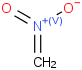138.9129.5± 1.2kJ/mol60.0321 ±
0.0010
16787-85-2*0
15.0C6H5N(O)O (g)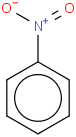81.461.4± 1.4kJ/mol123.1094 ±
0.0049
98-95-3*0
14.9C6H5N(O)O (cr,l)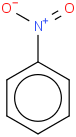6.3± 1.4kJ/mol123.1094 ±
0.0049
98-95-3*500
9.4HN(O)O (g)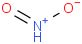-37.1-44.1± 1.5kJ/mol47.01348 ±
0.00061
90266-90-3*0
8.1H2O (cr, l, eq.press.)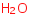-286.304-285.832± 0.026kJ/mol18.01528 ±
0.00033
7732-18-5*499
8.1H2O (cr,l)-286.302-285.830± 0.026kJ/mol18.01528 ±
0.00033
7732-18-5*500
8.1H2O (l, eq.press.)-285.832± 0.026kJ/mol18.01528 ±
0.00033
7732-18-5*589
8.1H2O (l)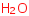-285.830± 0.026kJ/mol18.01528 ±
0.00033
7732-18-5*590
8.1[H3O]+ (aq)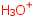-285.830± 0.026kJ/mol19.02267 ±
0.00037
13968-08-6*800

## Most Influential reactions involving CH3N(O)O (cr,l)

Please note: The list, which is based on a hat (projection) matrix analysis, is limited to no more than 20 largest influences.

Influence
Coefficient
TN
ID
Reaction Measured Quantity Reference
0.533CH3N(O)O (cr,l) + 3/2 O2 (g) → 2 CO2 (g) + 3 H2O (l) N2 (g) ΔrH°(298.15 K) = -339.02 ± 0.28 kcal/molProsen 1954, Cass 1958
0.261CH3N(O)O (cr,l) + 3/2 O2 (g) → 2 CO2 (g) + 3 H2O (l) N2 (g) ΔrH°(298.15 K) = -339.2 ± 0.4 kcal/molLebedeva 1973, note unc2
0.245ΔrH°(298.15 K) = 9.09 ± 0.09 kcal/molHolcomb 1949
0.245ΔrH°(298.15 K) = 9.17 ± 0.09 kcal/molJones 1947, est unc
0.245ΔrH°(298.15 K) = 9.171 ± 0.09 kcal/molMcCullough 1954, est unc
0.240ΔrH°(298.15 K) = 38.36 ± 0.38 kJ/molMajer 1985
0.041CH3N(O)O (cr,l) + 3/2 O2 (g) → 2 CO2 (g) + 3 H2O (l) N2 (g) ΔrH°(298.15 K) = -339.0 ± 1 kcal/molSwientoslawski 1910, est unc
0.016CH3N(O)O (cr,l) + 3/2 O2 (g) → 2 CO2 (g) + 3 H2O (l) N2 (g) ΔrH°(298.15 K) = -1425.02 ± 1.10 (×6.037) kJ/molVerevkin 2014
0.004CH3N(O)O (cr,l) + 3/2 O2 (g) → 2 CO2 (g) + 3 H2O (l) N2 (g) ΔrH°(298.15 K) = -336.0 ± 0.6 (×5.076) kcal/molKnobel 1971
0.000CH3N(O)O (cr,l) + 3/2 O2 (g) → 2 CO2 (g) + 3 H2O (l) N2 (g) ΔrH°(298.15 K) = -348.8 ± 0.6 (×16.35) kcal/molCass 1958
0.000CH3N(O)O (cr,l) + 3/2 O2 (g) → 2 CO2 (g) + 3 H2O (l) N2 (g) ΔrH°(298.15 K) = -350.49 ± 0.36 (×32) kcal/molHolcomb 1949

References
 1 B. Ruscic, R. E. Pinzon, M. L. Morton, G. von Laszewski, S. Bittner, S. G. Nijsure, K. A. Amin, M. Minkoff, and A. F. Wagner, Introduction to Active Thermochemical Tables: Several "Key" Enthalpies of Formation Revisited. J. Phys. Chem. A 108, 9979-9997 (2004) [DOI: 10.1021/jp047912y] 2 B. Ruscic, R. E. Pinzon, G. von Laszewski, D. Kodeboyina, A. Burcat, D. Leahy, D. Montoya, and A. F. Wagner, Active Thermochemical Tables: Thermochemistry for the 21st Century. J. Phys. Conf. Ser. 16, 561-570 (2005) [DOI: 10.1088/1742-6596/16/1/078] 3 B. Ruscic and D. H. Bross, Active Thermochemical Tables (ATcT) values based on ver. 1.122p of the Thermochemical Network (2020); available at ATcT.anl.gov 4 P. B. Changala, T. L. Nguyen, J. H. Baraban, G. B. Ellison, J. F. Stanton, D. H. Bross, and B. Ruscic, Active Thermochemical Tables: The Adiabatic Ionization Energy of Hydrogen Peroxide. J. Phys. Chem. A 121, 8799-8806 (2017) [DOI: 10.1021/acs.jpca.7b06221] (highlighted on the journal cover) 5 D. Feller, D. H. Bross, and B. Ruscic, Enthalpy of Formation of N2H4 (Hydrazine) Revisited. J. Phys. Chem. A 121, 6187-6198 (2017) [DOI: 10.1021/acs.jpca.7b06017] 6 B. Ruscic, Uncertainty Quantification in Thermochemistry, Benchmarking Electronic Structure Computations, and Active Thermochemical Tables. Int. J. Quantum Chem. 114, 1097-1101 (2014) [DOI: 10.1002/qua.24605]

Formula
The aggregate state is given in parentheses following the formula, such as: g - gas-phase, cr - crystal, l - liquid, etc.

Uncertainties
The listed uncertainties correspond to estimated 95% confidence limits, as customary in thermochemistry (see, for example, Ruscic ).
Note that an uncertainty of ± 0.000 kJ/mol indicates that the estimated uncertainty is < ± 0.0005 kJ/mol.

Website Functionality Credits
The reorganization of the website was developed and implemented by David H. Bross (ANL).
The find function is based on the complete Species Dictionary entries for the appropriate version of the ATcT TN.
The molecule images are rendered by Indigo-depict.
The XYZ renderings are based on Jmol: an open-source Java viewer for chemical structures in 3D. http://www.jmol.org/.

Acknowledgement
This work was supported by the U.S. Department of Energy, Office of Science, Office of Basic Energy Sciences, Division of Chemical Sciences, Geosciences and Biosciences under Contract No. DE-AC02-06CH11357.